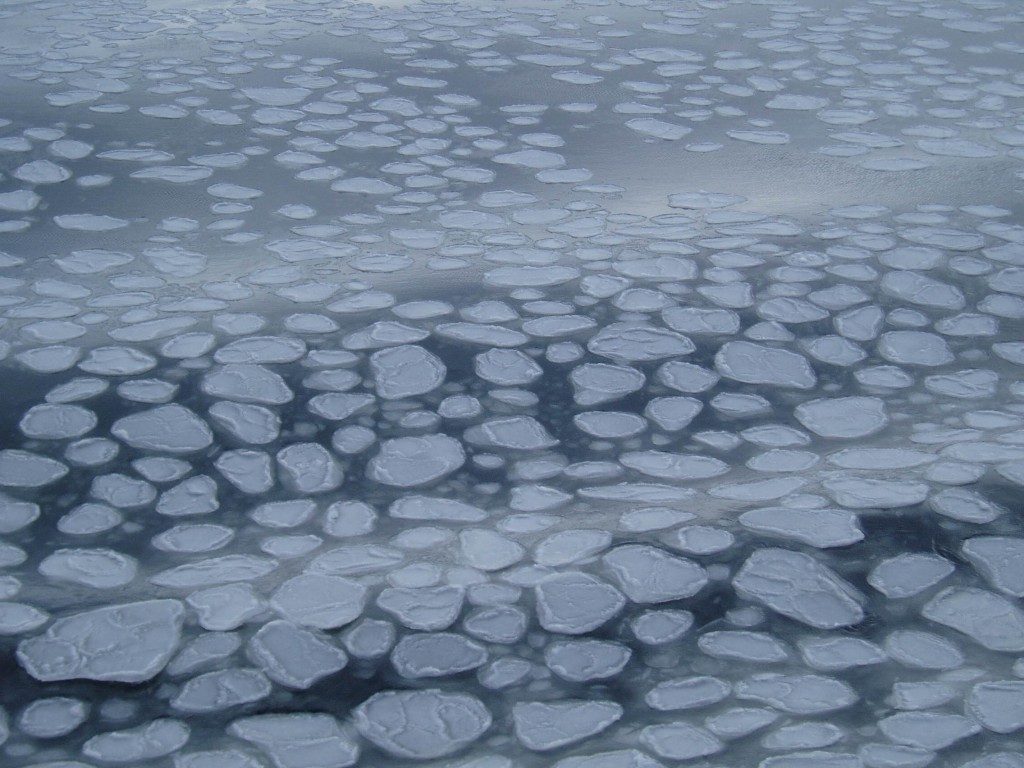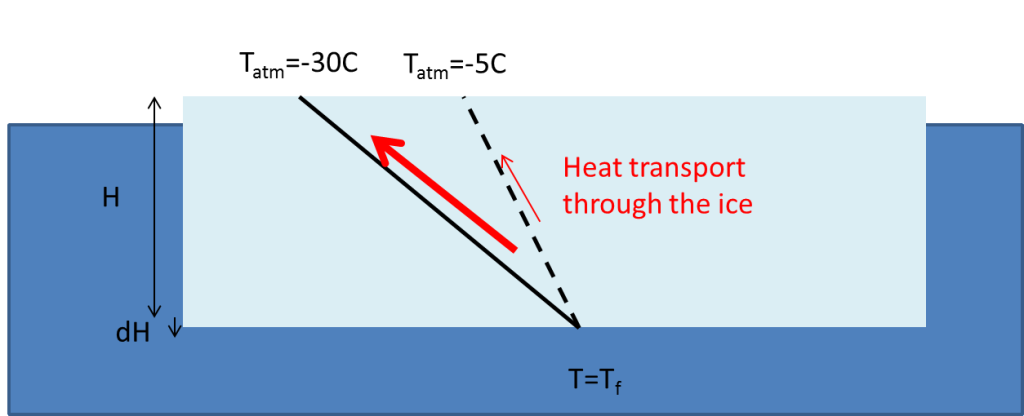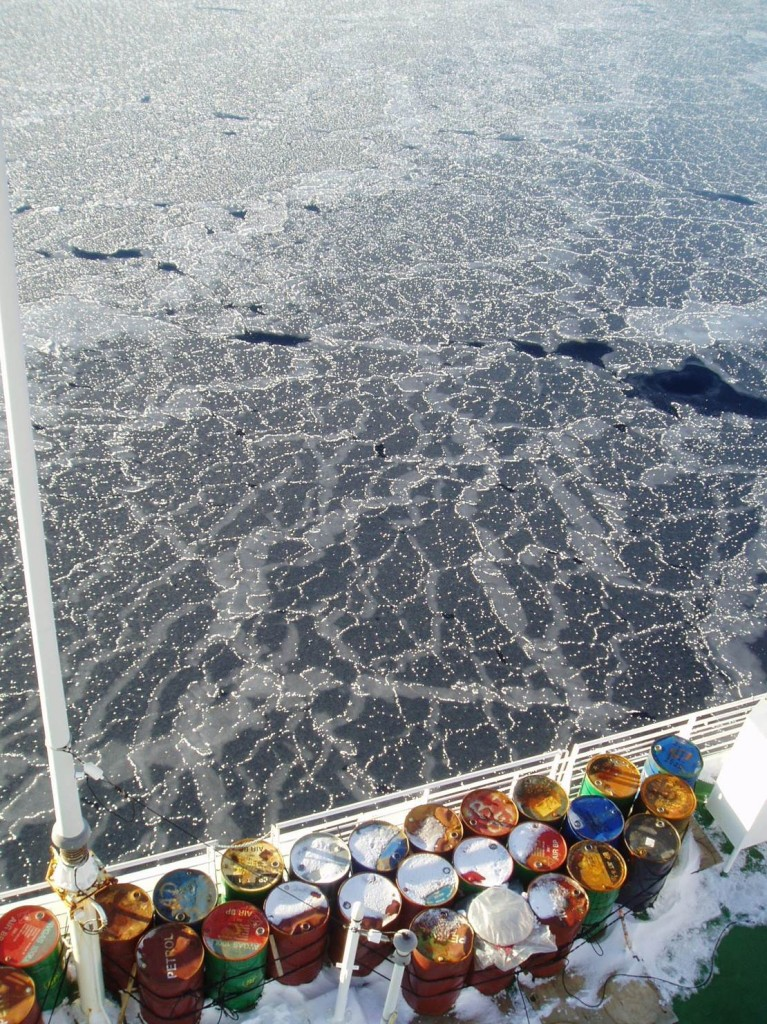# Exercises: IcePancake ice! If there are waves when the ice is freezing on the sea, you’ll typically see “pancake ice” being formed. Photo: Elin Darelius Chiche.

Ice, ice, ice! There is sea ice everywhere! Large floes, small floes and the odd iceberg. It is all white, and all beautiful, and it puts a definitive end to waves and seasickness!

When heat is removed from water, the water will cool and it will continue to do so until it reaches its freezingpoint. If we continue to remove heat, the water will freeze. The heat that we remove is then the latent heat. Normally it is not “we” who remove heat, but the atmosphere. When the air above is colder than the ocean below, heat will move from the water up into the air: the colder it is, the faster the heat moves. That is, the colder it is, the faster the ice grows. But the ice is a good isolator; it will isolate the water from the cold atmosphere above. Just like your jacket isolates you when it is cold outside and makes you keep the warmth inside, the ice causes the water to loose heat more slowly, since the heat must be conducted through the ice in order to be lost to the atmosphere. The thicker the ice is, the more slowly is the heat conducted through the ice, since the heatflux ($$F_{ice}$$, i.e. the amount of heat that is conducted through the ice per unit of time) is proportional to the temperature gradient:

$$F_{ice}=-k_{ice}\frac{dT}{dz}=-k_{ice}\frac{T_{atm}-T_{f}}{H}$$

$$k_{ice}=2\,W\,m^{-1}\,^{\circ}C^{-1}$$ is the heat conductivity of ice and $$H$$ is the thickness of the ice.

$$T_{atm}$$ is the temperature at the top of the ice (which we assume to be equal to the temperature of the air) and $$T_f=-1.9^\circ C$$ is the temperature at the bottom of the ice, that is, the freezingpoint of sea water.

The latent heat that is released (per square meter) when the ice is growing a little bit$$dH$$ is $$\rho_{ice}LdH$$. If that happens during a short time $$dt$$, then the latent heat flux is:

$$F_{latent}=\rho_{ice}L\frac{dH}{dt}$$

$$\rho_{ice}=900\,kg\,m^{-3}$$ is the density of ice andIce on the sea. The black lines show the temperature gradient in the ice and the red Arrows the heat flux. When the air above is cold (or if the ice is thin) the gradients and the heat flux is large and the ice grows quickly. When the temperature difference between the air and the water is small the gradient and the heat flux is small.

$$L=3.3*10^5J\,kg^{-1}$$ is the latent heat of fusion.

The ice will grow exactly as fast as the latent heat can be conducted up through the ice, i.e. so that

$$F_{latent}=F_{ice}$$

When combining the two equations we get a differential equation, that we can solve to get an expression for how the ice thickness increase in time, $$H(t)$$.

## Exercise 1

a) Set up the differential equation and show that the solution $$H(t)$$ is

$$H=\sqrt{ H_0^2+\frac{2k_{ice}(T_{f}-T_{atm})}{\rho_{is}L}t}$$.

when $$H(t=0)=H_0$$

Hint: Use the chain rule $$\frac{dH^2}{dt}=2H\frac{dH}{dt}$$.

b) Let $$H_0=0$$ and plot the function for different $$T_{atm}$$!When does the ice grow fastest? Why?

c) Use the equation from (a) to calculate the thickness of the ice ten hours after it started freezing if the temperature outside is (i) -20C (ii) -2C.

d) When the ice is 1 m thick, how long is it before it grow another 10cm?

e) What do you think will happen if there is snow falling on the ice? $$\kappa_{\textit{snow}}$$ is typically between 0.15 and 0.4$$W\,m^{-1}\,^{\circ}C^{-1}$$. What is the better isolator? Snow or ice?

f) All heat that is conducted up through the ice has to be conducted through the snow as well. Where is the temperature gradient largest? In the snow or in the ice? Make a sketch!

## Exercise 2

The temperature varies from day to day and from year to year. The file ENG_Temperatur gives temperature data from the Amundsen Sea from March 2014 to March 2015.

a) Find the mean temperature for each month and plot it. Find the standard deviation and add it to your graph. What month is coldest? Warmest? When is the temperature most variable?

b) When does the ice stop to grow?

c) Find out how much the ice thickness increase everyt month? What value should you use for $$H_0$$?

d) Plot the (i) the ice thickness and (ii) the ice growth as a function of time. When is the ice growing fastest? Is this when it is coldest? Why? Why not?If it is calm and no Wind when the ice freezes there are no pancakes but socalled “nilas”: thinice that looks almost black as one sees the dark open beneath. The thin icefloes can easily slide on top of ar below each other.

## Exercise 3

1. If an ice flow is 30 cm thick, 2 m wide and 5 m long – how much of the ice floe is then above water? $$\rho_{ice}=900kg m^{-3}$$
2. How many scientist can stand on the ice flow (in the middle)without getting their feet wet?
3. How much snow can fall on the ice before the ice floe is submerged? $$\rho_{snow}\approx 300kg m^{-3}$$

## Exercise 4

The ice in Antarctica is relatively thin and it often snows so much that the ice is submerged. Then we’ll have a layer of slush (snow + seawater) on top of the ice. When the slush freezes, we get what is often called “snow ice”. Estimations suggests that as much as 40% of the ice in the Amundsen Sea is snow ice!

1. It is quicker to freeze snow ice than regular sea ice – can you explain why? How far does the heat have to be conducted when freezing snow ice? Does the snow have to freeze?